# Step-by-step procedure for choosing a learning rate (and other optimization hyperparameters)

I’ve been using deep neural networks (DNNs) in my research. DNNs, as is often preached, are powerful models, capable of mapping almost any function. However, after the sermon is over, someone starting to train a DNN in the wild can quickly be overwhelmed by the many subtle technical details involved. The architecture, regularization techniques, and optimization are all inter-related, and these relationships change for different datasets.

My first steps in training a DNN primarily focused on architecture—do I have enough layers and filters for my problem? The optimization—stochastic gradient descent (SGD) with all of its hyperparameters (learning rate, momentum, …) and variants—was an afterthought, and I chose hyperparameters that seemed reasonable. Still, a nagging question persisted in the back of my mind: What if different hyperparameters led to even better performance? It was an obvious case of fear-of-missing-out (FOMO).

## Grid search (aka brute force) and black-box optimization techniques should be last resorts.

Due to FOMO, I began to more properly choose my hyperparameters by using a grid search (e.g., random search). This takes a lot of time. For each tweak of the architecture, I would need to rerun the entire grid search. For each grid search, out popped a solution and nothing else—no intuition about my problem, no understanding about the tradeoffs of the hyperparameters, and no clear way to check my code for bugs (except evaluating performance). The same can be said for black-box optimization techniques—which are fancier versions of grid search. I felt ashamed each grid search I ran because it’s brainless. It encourages me not to think about my data, model, or the bridge between the two: optimization.

## Let’s use our intuition about optimization to help guide our choice of hyperparameters.

Optimization is not a black-box method. In fact, gradient descent is one of the most intuitive concepts in math: You are a hiker on top of a mountain, and you need to get down. What direction and how far do you move? Inspired by this, I started reading about other ways to choose optimization hyperparameters. I came up with a step-by-step procedure that I now follow for every problem. It’s largely inspired by Smith 2018 and Jordan 2018.

This procedure outputs:
1) reasonable values for the hyperparameters,
2) intuition about the problem, and
3) red flags if there are bugs in your code.
The procedure takes a couple of training runs (fast!).

[Note: This procedure is great for researchers who want to get a model up and running. Grid/black-box search is more appropriate for architecture searches and when you really care about gaining 1% in accuracy.]

## Step-by-step procedure:

Here’s the full procedure. I’ll discuss each step individually.

1. Compute initial line search.
2. Increase learning rate.
3. Increase momentum.
4. Check if learning rate decay helps.
5. Check if a cyclical learning rate helps.
6. (optional) Check your other favorite SGD variants.
7. Compute final line search, for closure.

The procedure is general for any architecture and problem. For an example problem, I run the procedure on a small (3-layer) convolutional neural network to predict the responses of visual cortical neurons from image features (see Cowley and Pillow, 2020). I report accuracy on heldout data, as we want to make sure our optimized solution generalizes. The description of each step is short for ease of mind; I assume you are familiar with optimization and SGD (if you aren’t, check out the Convex Optimization book).

For a quick review, here is the equation for gradient descent: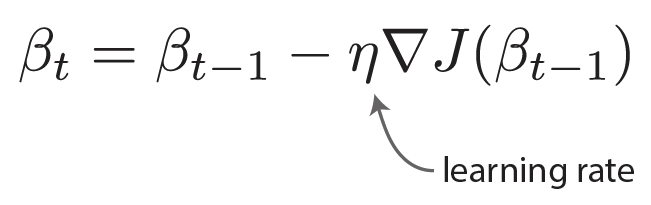And here are the equations for gradient descent with momentum: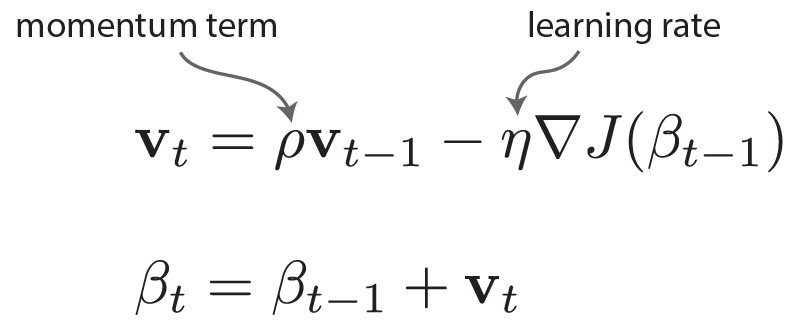## Step 1: Compute initial line search.

• Choose a direction in weight space (found either by training on a small amount of data or choosing weights randomly).
• Perform a line search by taking small steps (linear increments) along that direction, plotting accuracy for each step.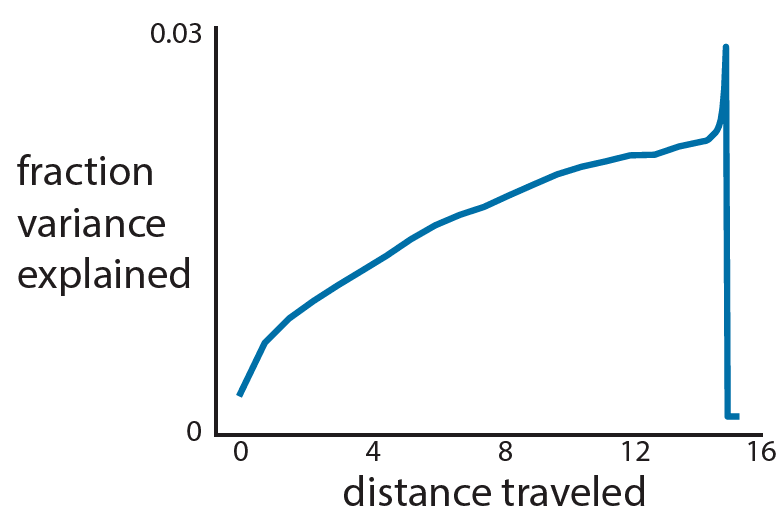• How nonconvex/complicated is your accuracy landscape? Here, I have a nonconvex problem with a meandering slope and a sharp ridge with a cliff.
→ Choosing a large learning rate may mean I fall off the cliff!

Step 2: Increase learning rate.

• Start with an epsilon-small learning rate. Then, increase the learning rate for each consecutive training epoch.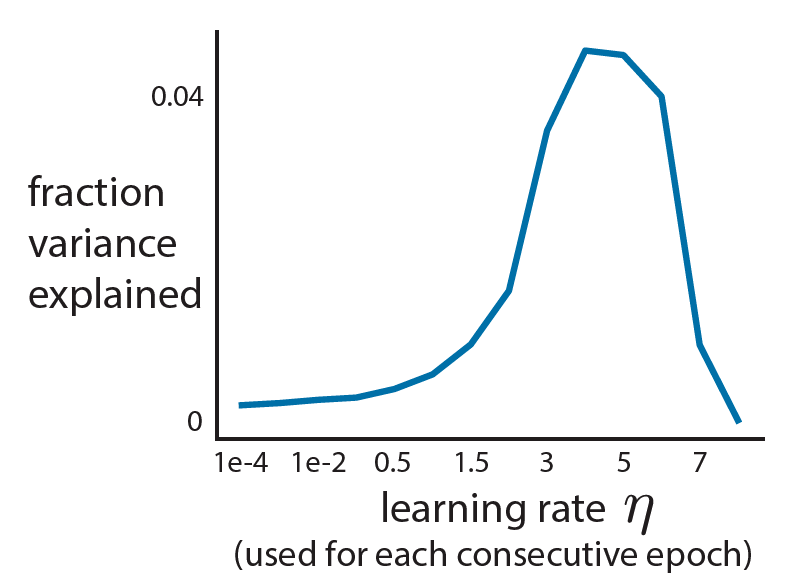• Small learning rates lead to small increases in accuracy.
→ You’ve barely moved in weight space.
• Large learning rates lead to large decreases in accuracy.
→ You’re moving too far, and you’ve jumped off the cliff!
• Just right learning rates are where the accuracy increases steadily.
• Here, we choose a learning rate of 1.5.

## Step 3: Increase momentum.

• Train for N epochs with the chosen learning rate.
• Similar to Step 2, start with a small momentum and increase it each training epoch.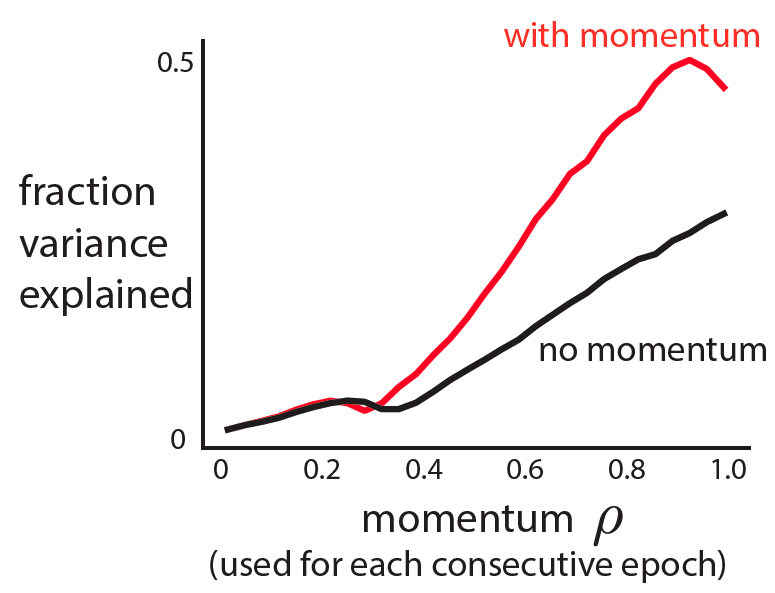• A small momentum yields only a small increase in accuracy.
A large momentum sees a drop in accuracy.
• Choose a momentum in the sweet spot—where accuracy is steadily increasing.
• Here, we choose a momentum of 0.7.
• Using the chosen learning rate and momentum, train for N epochs. Confirm that momentum improves performance.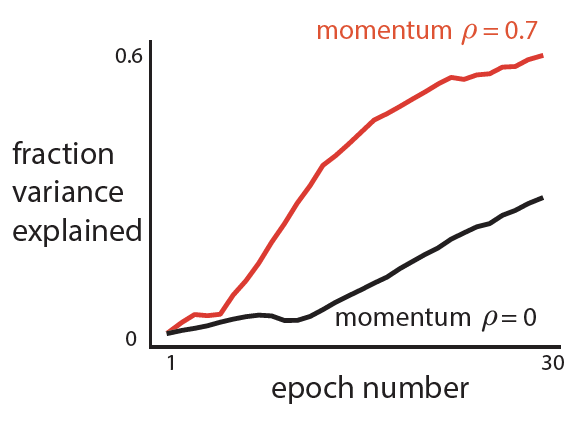• Thoughts: Momentum does help in this case (red above black).

## Step 4: Check if learning rate decay helps.

• Train model until convergence (might be longer than N epochs).
• Use a schedule for learning rate decay. The idea is that as you get closer to an optimum, you should make smaller steps (i.e., decay) to prevent yourself from jumping passed the optimum. Lots of choices here.
• My schedule is a power decay:
Let M be the epoch number where performance plateaus/falls off.
Then alpha = (⅔)^(1/M).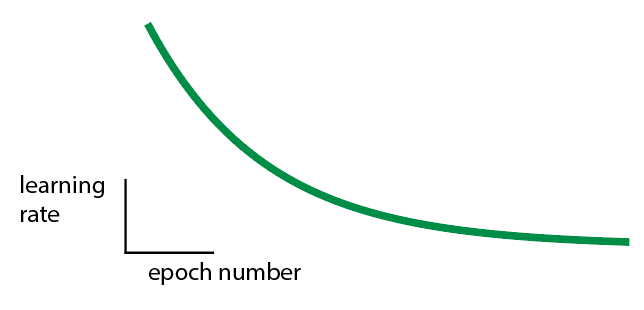• Train model with learning rate decay. Confirm learning rate decay is needed.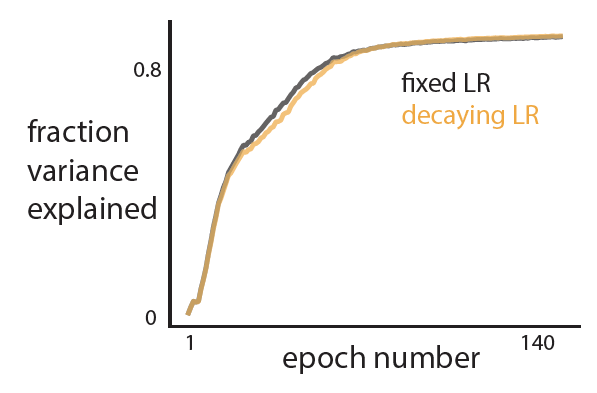• Thoughts: Learning rate decay is not really helpful for my problem. So I won’t use it.

## Step 5: Check if a cyclical learning rate helps.

• Use a cyclical learning rate. The idea is that you may want to explore different optima, so we need a way to push ourselves far from the current optimum.

Here’s one option:
• Choose the upper learning rate bound as 2 * chosen learning rate.
• Choose the lower learning rate bound as ½ * chosen learning rate.
• Start the learning rate at the upper bound then shrink to the lower bound after M epochs (where M is chosen from Step 4). Then, switch back to the largest learning rate: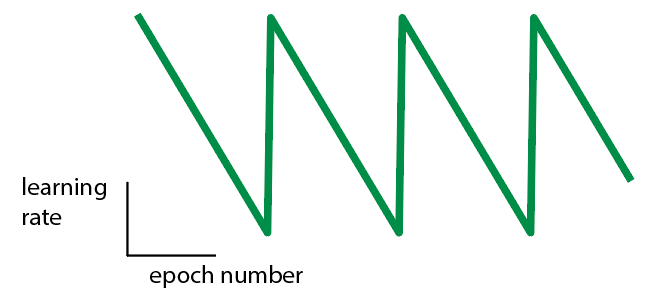• Train model with a cyclical learning rate. Confirm that a cyclical learning rate is needed.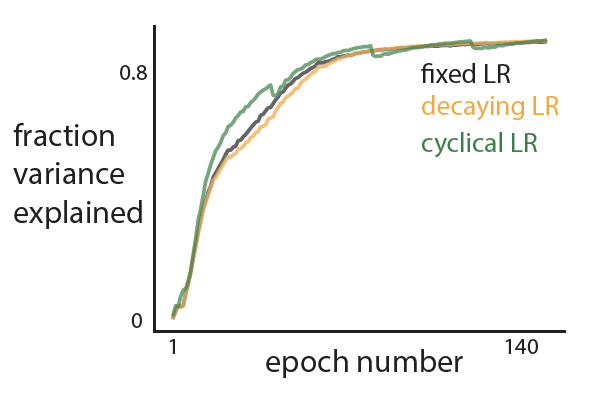• Thoughts: A cyclical learning rate does train faster, but not substantially. So I won’t use it.

## Step 6: (optional) Check your other favorite SGD variants.

• Likely everyone has their favorite SGD variant. Try it here!
Note: Don’t use the hyperparameters chosen above for some SGD variants, like Adam (which usually assumes a very small initial learning rate). Instead, you may need to repeat some steps to find the variant’s hyperparameters.
• I ran Adam on the same dataset, choosing a learning rate of 1-e2 (much smaller than the learning rate of 1.5 chosen in Step 1!).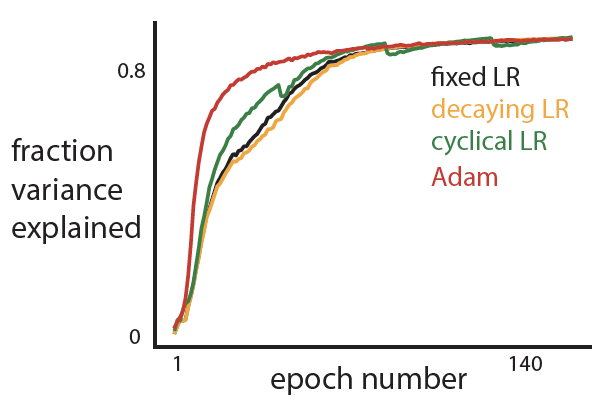• Adam does well in the beginning, likely because it adapts its learning rate and can get away with a large learning rate for the earlier epochs.
• I’ve found that Adam doesn’t always work well. This is especially true when training a DNN to predict behavior of different subjects—each subject’s behavior (e.g., velocity) may have a different variance. This is why we check!

## Step 7: Compute final line search, for closure.

• This is my favorite step. It’s the exact same as Step 1, except we now compute a line search in weight space along a line between the initial, untrained weight vector and the final, trained weight vector.• Thoughts: This looks very similar to Step 1. The optimization first finds the ridge and then moves along this ridge.
• In this example, there doesn’t seem to be multiple local minima. But this is not always the case!  Here’s a final line search from one of my other research projects:## Final thoughts

You can see why I like this procedure so much. It takes about an afternoon to run, and I get some interesting plots to look at and think about. The procedure outputs a nice set of hyperparameters, some intuition about the optimization problem we face, and gives me peace of mind that I shouldn’t keep twiddling hyperparameters and preventing my FOMO.

It would be great to have a gallery of observed final line searches (Step 7), just to see the variety of loss landscapes out there. In the meantime, you can check out these artistic renderings of loss landscapes: losslandscape.com